# Lect w13 152_electrochemistry_abbrev

1 de May de 2011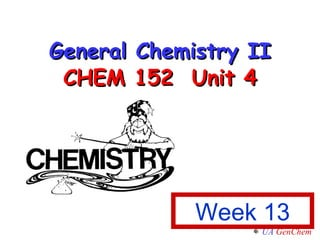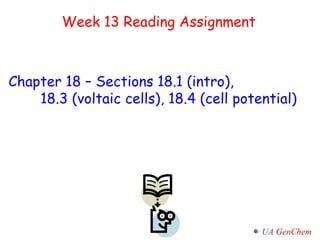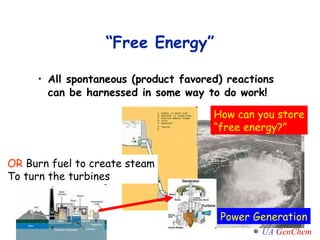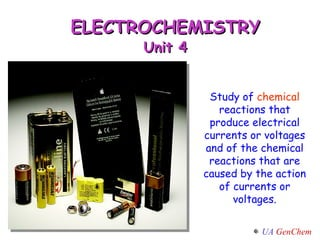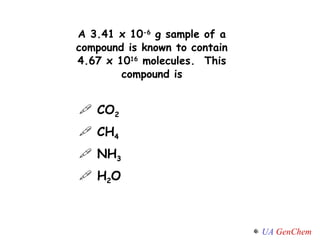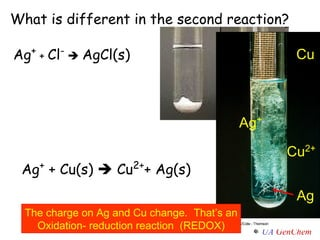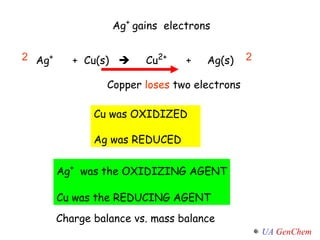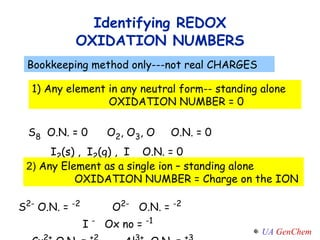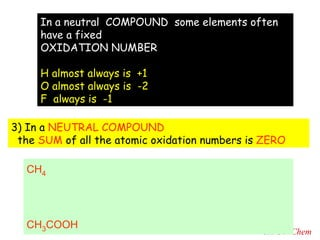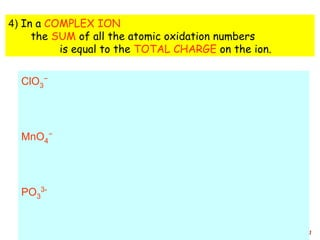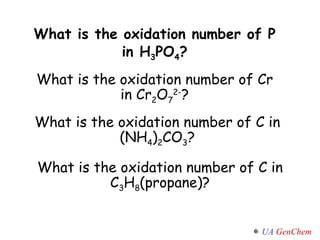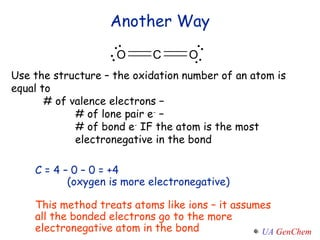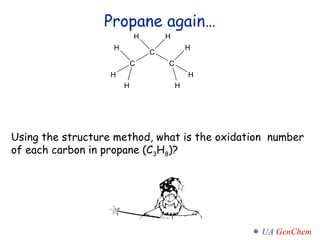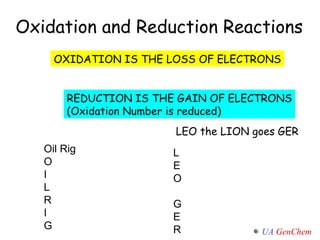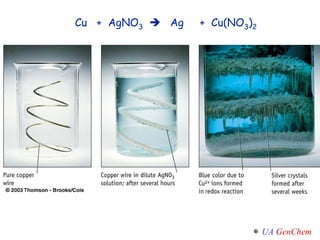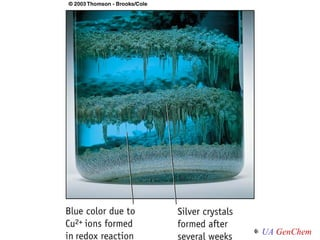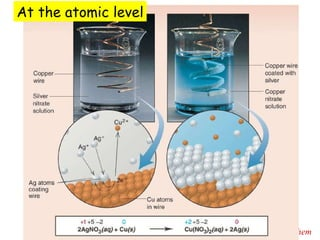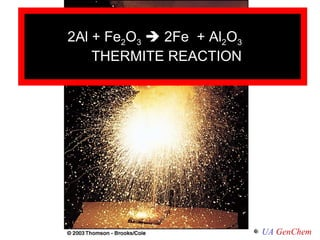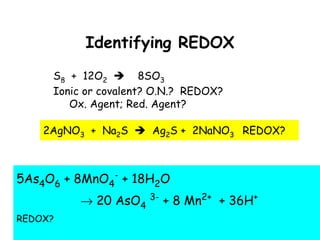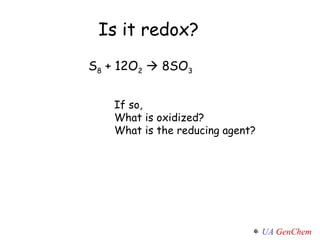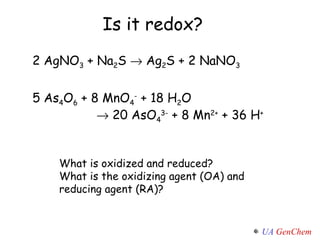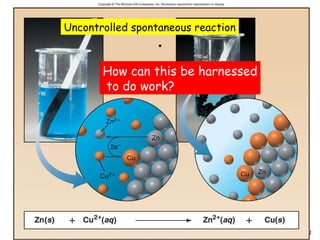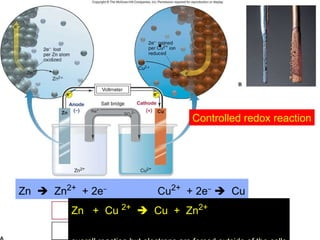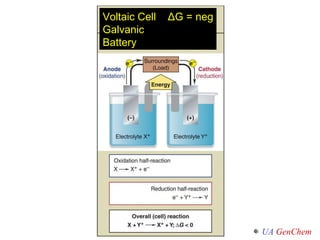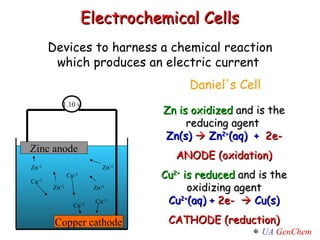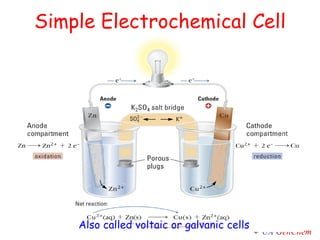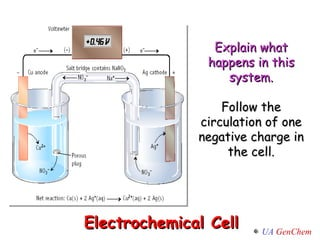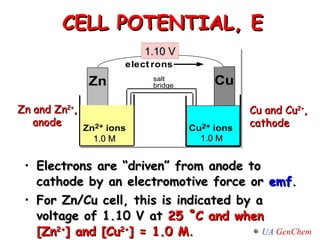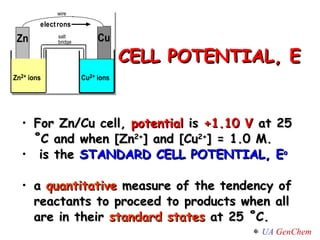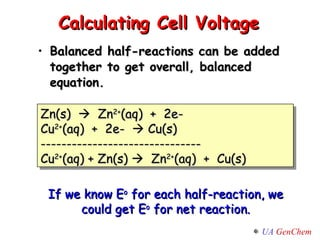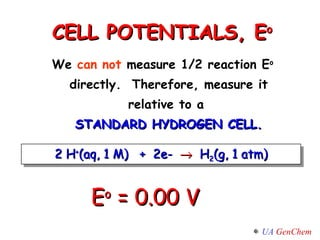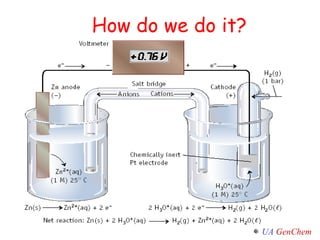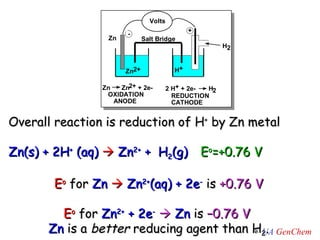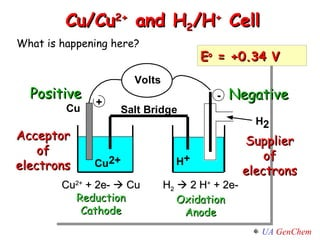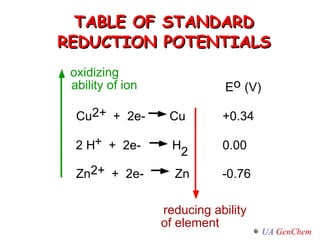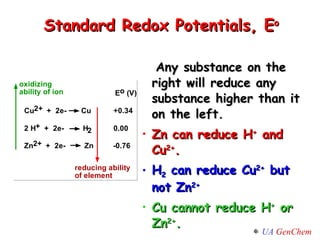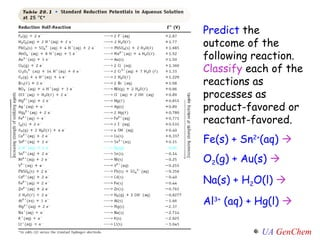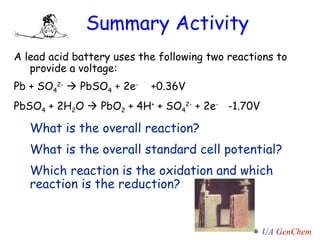1 de 40

### Lect w13 152_electrochemistry_abbrev

• 1. General Chemistry II CHEM 152 Unit 4 Week 13
• 2. Week 13 Reading Assignment Chapter 18 – Sections 18.1 (intro), 18.3 (voltaic cells), 18.4 (cell potential)
• 3.
• 4. ELECTROCHEMISTRY Unit 4 Study of chemical reactions that produce electrical currents or voltages and of the chemical reactions that are caused by the action of currents or voltages.
• 5.
• 6. What is different in the second reaction? Ag + + Cl -  AgCl(s) Ag + + Cu(s)  Cu 2+ + Ag(s) Cu Ag Ag + The charge on Ag and Cu change. That’s an Oxidation- reduction reaction (REDOX) Cu 2+
• 7. Ag + + Cu(s)  Cu 2+ + Ag(s) Copper loses two electrons Cu was OXIDIZED Ag was REDUCED Ag + was the OXIDIZING AGENT Cu was the REDUCING AGENT Charge balance vs. mass balance 2 2 Ag + gains electrons
• 8.
• 9. In a neutral COMPOUND some elements often have a fixed OXIDATION NUMBER H almost always is +1 O almost always is -2 F always is -1 3) In a NEUTRAL COMPOUND the SUM of all the atomic oxidation numbers is ZERO CH 4 CH 3 COOH
• 10. 4) In a COMPLEX ION the SUM of all the atomic oxidation numbers is equal to the TOTAL CHARGE on the ion. ClO 3 − MnO 4 − PO 3 3-
• 11. What is the oxidation number of P in H 3 PO 4 ? What is the oxidation number of Cr in Cr 2 O 7 2- ? What is the oxidation number of C in (NH 4 ) 2 CO 3 ? What is the oxidation number of C in C 3 H 8 (propane)?
• 12. Another Way Use the structure – the oxidation number of an atom is equal to # of valence electrons − # of lone pair e - − # of bond e - IF the atom is the most electronegative in the bond C = 4 – 0 – 0 = +4 (oxygen is more electronegative) This method treats atoms like ions – it assumes all the bonded electrons go to the more electronegative atom in the bond
• 13. Propane again… Using the structure method, what is the oxidation number of each carbon in propane (C 3 H 8 )?
• 14. What is the oxidation number of the oxygen-bonded C in CH 3 COOH (acetic acid)?
• 15. OXIDATION IS THE LOSS OF ELECTRONS REDUCTION IS THE GAIN OF ELECTRONS (Oxidation Number is reduced) Oil Rig O I L R I G Oxidation and Reduction Reactions LEO the LION goes GER L E O G E R
• 16. Cu + AgNO 3  Ag + Cu(NO 3 ) 2
• 17.
• 18. At the atomic level
• 19. 2Al + Fe 2 O 3  2Fe + Al 2 O 3 THERMITE REACTION
• 20.
• 21. Identifying REDOX S 8 + 12O 2  8SO 3 Ionic or covalent? O.N.? REDOX? Ox. Agent; Red. Agent? 2AgNO 3 + Na 2 S  Ag 2 S + 2NaNO 3 REDOX? 5As 4 O 6 + 8MnO 4 - + 18H 2 O  20 AsO 4 3- + 8 Mn 2+ + 36H + REDOX?
• 22. S 8 + 12O 2  8SO 3 Is it redox? If so, What is oxidized? What is the reducing agent?
• 23. 2 AgNO 3 + Na 2 S  Ag 2 S + 2 NaNO 3 Is it redox? 5 As 4 O 6 + 8 MnO 4 - + 18 H 2 O  20 AsO 4 3- + 8 Mn 2+ + 36 H + What is oxidized and reduced? What is the oxidizing agent (OA) and reducing agent (RA)?
• 24. . Uncontrolled spontaneous reaction How can this be harnessed to do work?
• 25. . Controlled redox reaction Zn  Zn 2+ + 2e − Cu 2+ + 2e −  Cu Zn + Cu 2+  Cu + Zn 2+ + overall reaction but electrons are forced outside of the cells
• 26. Voltaic Cell Δ G = neg Galvanic Battery
• 27. Electrochemical Cells Devices to harness a chemical reaction which produces an electric current Daniel's Cell Zn is oxidized and is the reducing agent Zn(s)  Zn 2+ (aq) + 2e- ANODE (oxidation) Cu 2+ is reduced and is the oxidizing agent Cu 2+ (aq) + 2e-  Cu(s) CATHODE (reduction) Zinc anode Copper cathode Cu +2 Cu +2 Cu +2 Cu +2 1.10 v Zn +2 Zn +2 Zn +2 Zn +2
• 28. Simple Electrochemical Cell Also called voltaic or galvanic cells
• 29. Electrochemical Cell Explain what happens in this system. Follow the circulation of one negative charge in the cell.
• 30.
• 31.
• 32.
• 33.
• 34. How do we do it?
• 35. Overall reaction is reduction of H + by Zn metal Zn(s) + 2H + (aq)  Zn 2+ + H 2 (g) E o =+0.76 V E o for Zn  Zn 2+ (aq) + 2e - is +0.76 V E o for Zn 2+ + 2e -  Zn is –0.76 V Zn is a better reducing agent than H 2 .
• 36.
• 37. TABLE OF STANDARD REDUCTION POTENTIALS 2 E o (V) Cu 2+ + 2e- Cu +0.34 2 H + + 2e- H 0.00 Zn 2+ + 2e- Zn -0.76 oxidizing ability of ion reducing ability of element
• 38.
• 39. Predict the outcome of the following reaction. Classify each of the reactions as processes as product-favored or reactant-favored. Fe(s) + Sn 2+ (aq)  O 2 (g) + Au(s)  Na(s) + H 2 O(l)  Al 3+ (aq) + Hg(l) 
• 40. Summary Activity A lead acid battery uses the following two reactions to provide a voltage: Pb + SO 4 2-  PbSO 4 + 2e -    +0.36V PbSO 4 + 2H 2 O  PbO 2 + 4H + + SO 4 2- + 2e -   -1.70V What is the overall reaction? What is the overall standard cell potential? Which reaction is the oxidation and which reaction is the reduction?

### Notas do Editor

1. Update for Tro.